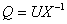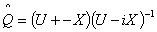The Australian Journal of Mathematical Analysis and Applications

 Home News Editors Volumes RGMIA Subscriptions Authors Contact

ISSN 1449-5910

You searched for bohner
Total of 49 results found in site

16: Paper Source PDF document

Paper's Title:

On Oscillation of Second-Order Delay Dynamic Equations on Time Scales

Author(s):

S. H. Saker

Department of Mathematics, Faculty of Science,
Mansoura University, Mansoura, 35516,
Egypt.
shsaker@mans.edu.eg

Abstract:

Some new oscillation criteria for second-order linear delay dynamic equation on a time scale T are established. Our results improve the recent results for delay dynamic equations and in the special case when T=R, the results include the oscillation results established by Hille [1948, Trans. Amer. Math. Soc. 64 (1948), 234-252] and Erbe [Canad. Math. Bull. 16 (1973), 49-56.] for differential equations. When T=Z the results include and improve some oscillation criteria for difference equations. When T=hZ, h>0, T=qN and T=N2, i.e., for generalized second order delay difference equations our results are essentially new and can be applied on different types of time scales. An example is considered to illustrate the main results.

9: Paper Source PDF document

Paper's Title:

Oscillation and Boundedness of Solutions to First and Second Order Forced Dynamic Equations with Mixed Nonlinearities

Author(s):

Ravi P. Agarwal and Martin Bohner

Department of Mathematical Sciences, Florida Institute of Technology
Melbourne, FL 32901,
U.S.A.
bohner@mst.edu
URL:http://web.mst.edu/~bohner

Department of Economics and Finance, Missouri University of Science and Technology
Rolla, MO 65401,
U.S.A.
agarwal@fit.edu

Abstract:

Some oscillation and boundedness criteria for solutions to certain first and second order forced dynamic equations with mixed nonlinearities are established. The main tool in the proofs is an inequality due to Hardy, Littlewood and Pólya. The obtained results can be applied to differential equations, difference equations and q-difference equations. The results are illustrated with numerous examples.

6: Paper Source PDF document

Paper's Title:

The Riemann-Stieltjes Integral on Time Scales

Author(s):

D. Mozyrska, E. Pawłuszewicz, D. Torres

Faculty Of Computer Science,
Białystok University Of Technology,
15-351 Białystok,
Poland
d.mozyrska@pb.edu.pl

Department Of Mathematics,
University Of Aveiro,
3810-193 Aveiro,
Portugal
ewa@ua.pt

Department Of Mathematics,
University Of Aveiro,
3810-193 Aveiro,
Portugal
delfim@ua.pt

Abstract:

We study the process of integration on time scales in the sense of Riemann-Stieltjes. Analogues of the classical properties are proved for a generic time scale, and examples are given

4: Paper Source PDF document

Paper's Title:

Comparison Results for Solutions of Time Scale Matrix Riccati Equations and Inequalities

Author(s):

R. Hilscher

Department of Mathematical Analysis, Faculty of Science,
Masaryk University, Jan
áčkovo nám. 2a,
CZ-60200, Brno, Czech Republic.
hilscher@math.muni.cz
URL: http://www.math.muni.cz/~hilscher/

Abstract:

In this paper we derive comparison results for Hermitian solutions of time scale matrix Riccati equations and Riccati inequalities. Such solutions arise from special conjoined bases (X,U) of the corresponding time scale symplectic system via the Riccati quotient. We also discuss properties of a unitary matrix solutionof a certain associated Riccati equation.

3: Paper Source PDF document

Paper's Title:

Regular Variation on Time Scales and Dynamic Equations

Author(s):

Pavel Řehák

Institute of Mathematics, Academy of Sciences of the Czech Republic
Žižkova 22, CZ61662 Brno,
Czech Republic
rehak@math.muni.cz
URL:http://www.math.muni.cz/~rehak

Abstract:

The purpose of this paper is twofold. First, we want to initiate a study of regular variation on time scales by introducing this concept in such a way that it unifies and extends well studied continuous and discrete cases. Some basic properties of regularly varying functions on time scales will be established as well. Second, we give conditions under which certain solutions of linear second order dynamic equations are regularly varying. Open problems and possible directions for a future research are discussed, too.

3: Paper Source PDF document

Paper's Title:

Ostrowski Type Inequalities for Lebesgue Integral: a Survey of Recent Results

Author(s):

Sever S. Dragomir1,2

1Mathematics, School of Engineering & Science
Victoria University, PO Box 14428
Melbourne City, MC 8001,
Australia
E-mail: sever.dragomir@vu.edu.au

2DST-NRF Centre of Excellence in the Mathematical and Statistical Sciences,
School of Computer Science & Applied Mathematics,
University of the Witwatersrand,
Private Bag 3, Johannesburg 2050,
South Africa
URL: http://rgmia.org/dragomir

Abstract:

The main aim of this survey is to present recent results concerning Ostrowski type inequalities for the Lebesgue integral of various classes of complex and real-valued functions. The survey is intended for use by both researchers in various fields of Classical and Modern Analysis and Mathematical Inequalities and their Applications, domains which have grown exponentially in the last decade, as well as by postgraduate students and scientists applying inequalities in their specific areas.

3: Paper Source PDF document

Paper's Title:

Generalized Weighted Trapezoid and Grüss Type Inequalities on Time Scales

Author(s):

Eze Raymond Nwaeze

Department of Mathematics,
Tuskegee University,
Tuskegee, AL 36088,
USA.
E-mail: enwaeze@mytu.tuskegee.edu

Abstract:

In this work, we obtain some new generalized weighted trapezoid and Grüss type inequalities on time scales for parameter functions. Our results give a broader generalization of the results due to Pachpatte in . In addition, the continuous and discrete cases are also considered from which, other results are obtained.

2: Paper Source PDF document

Paper's Title:

Positive Periodic Time-Scale Solutions for Functional Dynamic Equations

Author(s):

Douglas R. Anderson and Joan Hoffacker

Department of Mathematics and Computer Science
Concordia College
andersod@cord.edu
URL:
http://www.cord.edu/faculty/andersod/

Department of Mathematical Sciences
Clemson University
Clemson, SC 29634 USA
johoff@clemson.edu
URL:
http://www.math.clemson.edu/facstaff/johoff.htm

Abstract:

Using Krasnoselskii's fixed point theorem, we establish the existence of positive periodic solutions to two pairs of related nonautonomous functional delta dynamic equations on periodic time scales, and then extend the discussion to higher-dimensional equations. Two pairs of corresponding nabla equations are also provided in an analogous manner.

2: Paper Source PDF document

Paper's Title:

Positive Solutions to a System of Boundary Value Problems for Higher-Dimensional Dynamic Equations on Time Scales

Author(s):

I. Y. Karaca

Department of Mathematics,
Ege University,
35100 Bornova, Izmir,
Turkey

ilkay.karaca@ege.edu.tr

URL: http://ege.edu.tr

Abstract:

In this paper, we consider the system of boundary value problems for higher-dimensional dynamic equations on time scales. We establish criteria for the existence of at least one or two positive solutions. We shall also obtain criteria which lead to nonexistence of positive solutions. Examples applying our results are also given.

1: Paper Source PDF document

Paper's Title:

Some Properties of the Solution of a Second Order Elliptic Abstract Differential Equation

Author(s):

A. Aibeche and K. Laidoune

Mathematics Department, Faculty of Sciences, University Ferhat Abbas, Setif,
Route de Scipion, 19000, Setif,
Algeria
aibeche@univ-setif.dz

Abstract:

In this paper we study a class of non regular boundary value problems for elliptic differential-operator equation of second order with an operator in boundary conditions. We give conditions which guarantee the coerciveness of the solution of the considered problem, the completeness of system of root vectors in Banach-valued functions spaces and we establish the Abel basis property of this system in Hilbert spaces. Finally, we apply this abstract results to a partial differential equation in cylindrical domain.

Search and serve lasted 1 second(s).